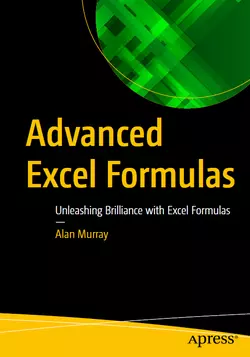### eBook Details:

• Paperback: 846 pages
• Publisher: WOW! eBook (August 29, 2022)
• Language: English
• ISBN-10: 1484271246
• ISBN-13: 978-1484271247

### Advanced Excel Formulas: Unleashing Brilliance with Excel Formulas

Enhance and upgrade your Excel knowledge with this comprehensive guide to formulas in Excel. Over 150 of the most useful Excel functions are covered with numerous practical examples of their use. This book is fully updated and includes examples of the most recently released functions in 2022. It is written in a manner that you can read it cover-to-cover or pick it up and learn something new in just 5 minutes.

The Advanced Excel Formulas book begins with a primer on Excel formulas and functions. Starting from a basic level, but diving into intricate detail and building a solid understanding of the fundamentals. It may not always be sexy, but learning why, in addition to how, is important to truly understanding Excel formulas. Having strong fundamentals is the most advanced skill.

The book covers all the most popular functions in Excel including VLOOKUP, SUMPRODUCT, COUNTIFS, MATCH, SORT and so much more. You will learn how to return multiple results with a single formula. Harness the power of the dynamic array engine and functions such as SEQUENCE, SORTBY, UNIQUE, XLOOKUP and FILTER to create top N Lists, models and reports that would seem impossible without array formulas. In the final chapter, we discover the amazing LAMBDA function in Excel. Create your own Excel functions to simplify future Excel formulas and share them with others.

• Gain intimate knowledge of Excel formulas
• Understand efficient and practical use of Defined Names and Tables
• Master the most popular functions of Excel – VLOOKUP, COUNTIFS, MATCH, SUMPRODUCT, and so much more
• Learn to return multiple results from a single formula with the magical array formulas.
• Know the best lookup functions for dynamic spreadsheets
• Know true power of classic Excel functions such as IF, SUM, and INDEX
• Use new Excel functions including XLOOKUP, VSTACK, LET, and LAMBDA

In this Advanced Excel Formulas book, the formulas are not only written to return values to the grid, but also for use with other Excel features such as charts and Conditional Formatting, to take them to another level. Practice files are provided to follow all examples shown in the book.